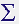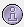# Percentage of classes

Hello everybody!
I created two masks of a rainforest area. The left scene was taken three years before the right one.
The green areas represent rainforest, the black ones are deforested regions.
I want to measure how many percent of the picture are covered by rainforest and how many are logged already. How can I measure the percentage of each class covering the total scene?

when the mask consists of 1 (forest) and 0 (non-forest) you can open the statisticstool and calculate the sum of all pixel values.
Then you calculate the total number of pixels in the infotool by multiplying rows and colums. The sum of pixel values divided by the total number of pixels is the percentage of forest cover.

Thak you very much!
It finally worked as I followed your guidance!